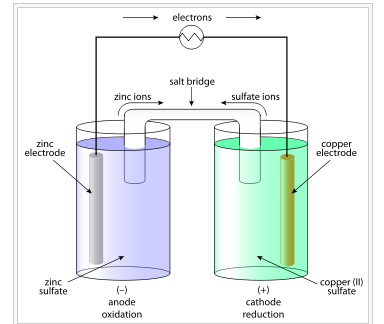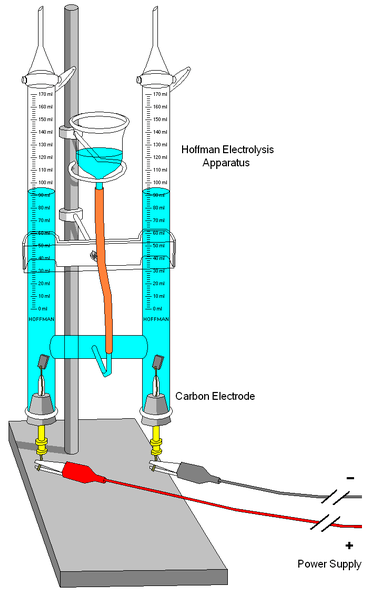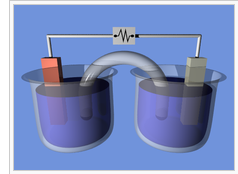## Voltaic Cells

A voltaic cell is a device that produces an electric current from energy released by a spontaneous redox reaction in two half-cells.

### Learning Objectives

Recall that reduction happens at the cathode and oxidation happens at the anode in a voltaic cell

### Key Takeaways

#### Key Points

• Oxidation describes the loss of electrons by a molecule, atom, or ion.
• Reduction describes the gain of electrons by a molecule, atom, or ion.
• The electrons always flow from the anode to the cathode.
• The half-cells are connected by a salt bridge that allows the ions in the solution to move from one half-cell to the other, so that the reaction can continue.

#### Key Terms

• redox: A reversible chemical reaction in which one reaction is an oxidation and the reverse is a reduction.
• half-cell: Either of the two parts of an electrochemical cell containing an electrode and an electrolyte.
• voltaic cell: A cell, such as in a battery, in which an irreversible chemical reaction generates electricity; a cell that cannot be recharged.

An electrochemical cell is a device that produces an electric current from energy released by a spontaneous redox reaction. This kind of cell includes the galvanic, or voltaic, cell, named after Luigi Galvani and Alessandro Volta. These scientists conducted several experiments on chemical reactions and electric current during the late 18th century.

Electrochemical cells have two conductive electrodes, called the anode and the cathode. The anode is defined as the electrode where oxidation occurs. The cathode is the electrode where reduction takes place. Electrodes can be made from any sufficiently conductive materials, such as metals, semiconductors, graphite, and even conductive polymers. In between these electrodes is the electrolyte, which contains ions that can freely move.

The voltaic cell uses two different metal electrodes, each in an electrolyte solution. The anode will undergo oxidation and the cathode will undergo reduction. The metal of the anode will oxidize, going from an oxidation state of 0 (in the solid form) to a positive oxidation state, and it will become an ion. At the cathode, the metal ion in the solution will accept one or more electrons from the cathode, and the ion’s oxidation state will reduce to 0. This forms a solid metal that deposits on the cathode. The two electrodes must be electrically connected to each other, allowing for a flow of electrons that leave the metal of the anode and flow through this connection to the ions at the surface of the cathode. This flow of electrons is an electrical current that can be used to do work, such as turn a motor or power a light.

### Example Reaction

The operating principle of the voltaic cell is a simultaneous oxidation and reduction reaction, called a redox reaction. This redox reaction consists of two half-reactions. In a typical voltaic cell, the redox pair is copper and zinc, represented in the following half-cell reactions:

Zinc electrode (anode): Zn(s) → Zn2+(aq) + 2 e

Copper electrode (cathode): Cu2+(aq) + 2 e → Cu(s)

The cells are constructed in separate beakers. The metal electrodes are immersed in electrolyte solutions. Each half-cell is connected by a salt bridge, which allows for the free transport of ionic species between the two cells. When the circuit is complete, the current flows and the cell “produces” electrical energy.A galvanic, or voltaic, cell: The cell consists of two half-cells connected via a salt bridge or permeable membrane. The electrodes are immersed in electrolyte solutions and connected through an electrical load.

Copper readily oxidizes zinc; the anode is zinc and the cathode is copper. The anions in the solutions are sulfates of the respective metals. When an electrically conducting device connects the electrodes, the electrochemical reaction is:

Zn + Cu2+ → Zn2+ + Cu

The zinc electrode produces two electrons as it is oxidized ($\text{Zn} \rightarrow \text{Zn}^{2+} + 2\text{e}^-$), which travel through the wire to the copper cathode. The electrons then find the Cu2+ in solution and the copper is reduced to copper metal ($\text{Cu}^{2+} + 2\text{e}^- \rightarrow \text{Cu}$). During the reaction, the zinc electrode will be used and the metal will shrink in size, while the copper electrode will become larger due to the deposited Cu that is being produced. A salt bridge is necessary to keep the charge flowing through the cell. Without a salt bridge, the electrons produced at the anode would build up at the cathode and the reaction would stop running.

Voltaic cells are typically used as a source of electrical power. By their nature, they produce direct current. A battery is a set of voltaic cells that are connected in parallel. For instance, a lead–acid battery has cells with the anodes composed of lead and cathodes composed of lead dioxide.

## Electrolytic Cells

Electrolysis uses electrical energy to induce a chemical reaction, which then takes place in an electrolytic cell.

### Learning Objectives

Recall the three components necessary to construct an electrolytic cell

### Key Takeaways

#### Key Points

• Electrometallurgy is the process of reducing metals from metallic compounds to obtain the pure form of the metal using electrolysis.
• Electrolysis can sometimes be thought of as running a non-spontaneous galvanic cell.
• Electrodes of metal, graphite, and semiconductor material are widely used in electrolysis.
• Other systems that utilize the electrolytic process are used to produce metallic sodium and potassium, chlorine gas, sodium hydroxide, and potassium and sodium chlorate.

#### Key Terms

• electrolysis: The chemical change produced by passing an electric current through a conducting solution or a molten salt.
• electrolytic: Of, relating to, or using electrolysis.

In chemistry and manufacturing, electrolysis is a method of using a direct electric current (DC) to drive an otherwise non-spontaneous chemical reaction. Electrolysis is commercially important as a stage in the process of separating elements from naturally occurring sources such as ore.

Electrolysis is the passage of a direct electric current through an ionic substance that is either molten or dissolved in a suitable solvent, resulting in chemical reactions at the electrodes and separation of the materials.

Electrolysis can sometimes be thought of as running a non-spontaneous galvanic cell. Depending on how freely elements give up electrons (oxidation) and how energetically favorable it is for elements to receive electrons (reduction), the reaction may not be spontaneous. By externally supplying the energy to overcome the energy barrier to spontaneous reaction, the desired reaction is “allowed” to run under special circumstances.

The main components required to perform electrolysis are:

• An electrolyte: a substance containing free ions that carry electric current. If the ions are not mobile, as in a solid salt, then electrolysis cannot occur.
• A direct current (DC) supply: provides the energy necessary to create or discharge the ions in the electrolyte. Electric current is carried by electrons in the external circuit.
• Two electrodes: an electrical conductor that provides the physical interface between the electrical circuit providing the energy and the electrolyte.A typical electrolysis cell: A cell used in elementary chemical experiments to produce gas as a reaction product and to measure its volume.

Electrodes of metal, graphite, and semiconductor material are widely used. Choosing a suitable electrode depends on the chemical reactivity between the electrode and electrolyte, and the cost of manufacture.

Other systems that utilize the electrolytic process are used to produce metallic sodium and potassium, chlorine gas, sodium hydroxide, and potassium and sodium chlorate.

## Electrochemical Cell Notation

Cell notation is shorthand that expresses a certain reaction in an electrochemical cell.

### Learning Objectives

Produce the appropriate electrochemical cell notation for a given electrochemical reaction

### Key Takeaways

#### Key Points

• The cell anode and cathode ( half-cells ) are separated by two bars or slashes, which represent a salt bridge.
• The anode is placed on the left and the cathode is placed on the right.
• Individual solid, liquid, or aqueous phases within each half-cell are written separated by a single bar.
• Concentrations of dissolved species can be written in the parentheses after the phase notation (s, l, g, or aq).

#### Key Terms

• half-cell: Either of the two parts of an electrochemical cell containing an electrode and an electrolyte.
• electrode: The terminal through which electric current passes between metallic and nonmetallic parts of an electric circuit. In electrolysis, the electrodes are placed in the solution separately.

### Cell Notation

Recall that standard cell potentials can be calculated from potentials E0cell for both oxidation and reduction reactions. A positive cell potential indicates that the reaction proceeds spontaneously in the direction in which the reaction is written. Conversely, a reaction with a negative cell potential proceeds spontaneously in the reverse direction.

$\text{E}^\text{o} _{\text{cell}} = \text{E}^\text{o} _{\text{reduction}} + \text{E}^\text{o} _{\text{oxidation}}$

Cell notations are a shorthand description of voltaic or galvanic (spontaneous) cells. The reaction conditions ( pressure, temperature, concentration, etc.), the anode, the cathode, and the electrode components are all described in this unique shorthand.

Recall that oxidation takes place at the anode and reduction takes place at the cathode. When the anode and cathode are connected by a wire, electrons flow from anode to cathode.A typical galvanic cell: A typical arrangement of half-cells linked to form a galvanic cell.

Using the arrangement of components, let’s put a cell together.

One beaker contains 0.15 M Cd(NO3)2 and a Cd metal electrode. The other beaker contains 0.20 M AgNO3 and a Ag metal electrode. The net ionic equation for the reaction is written:

$2\text{Ag}^+ (\text{aq}) + \text{Cd} (\text{s}) \rightleftharpoons \text{Cd}^{2+} (\text{aq}) + 2\text{Ag} (\text{s})$

In the reaction, the silver ion is reduced by gaining an electron, and solid Ag is the cathode. The cadmium is oxidized by losing electrons, and solid Cd is the anode.

The anode reaction is:

$\text{Cd} (\text{s}) \rightleftharpoons \text{Cd}^{2+} (\text{aq}) + 2\text{e}^-$

The cathode reaction is:

$2\text{Ag}^+ (\text{aq}) + 2\text{e}^- \rightleftharpoons 2\text{Ag} (\text{s})$

### Cell Notation Rules

1. The anode half-cell is described first; the cathode half-cell follows. Within a given half-cell, the reactants are specified first and the products last. The description of the oxidation reaction is first, and the reduction reaction is last; when you read it, your eyes move in the direction of electron flow. Spectator ions are not included.

2. A single vertical line ( | ) is drawn between two chemical species that are in different phases but in physical contact with each other (e.g., solid electrode | liquid with electrolyte ). A double vertical line ( || ) represents a salt bridge or porous membrane separating the individual half-cells.

3. The phase of each chemical (s, l, g, aq) is shown in parentheses. If the electrolytes in the cells are not at standard conditions, concentrations and/or pressure, they are included in parentheses with the phase notation. If no concentration or pressure is noted, the electrolytes in the cells are assumed to be at standard conditions (1.00 M or 1.00 atm and 298 K).

Using these rules, the notation for the cell we put together is:

Cd (s) | Cd2+ (aq, 0.15 M) || Ag+ (aq, 0.20 M) | Ag (s)